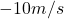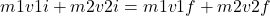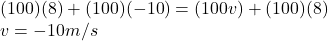## Two 100kg bumper cars are moving towards eachother in oppisite directions. Car A is moving at 8 m/s and Car B at -10 m/s when they collide h

Question

Two 100kg bumper cars are moving towards eachother in oppisite directions. Car A is moving at 8 m/s and Car B at -10 m/s when they collide head on. If the resulting velocity of Car B after the collision is 8 m/s, what is the velocity of Car A after the collision

in progress 0
2 months 2021-07-30T05:01:02+00:00 1 Answers 1 views 0Explanation:

When two cars collide then the momentum of two cars will remains conserved

• Mass of two cars = 100 kg
• Speed of car A = 8 m/s
• Speed of car B = – 10 m/s
• After collision the speed of car B = +8 m/s

By momentum conservation equation